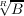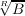#### Vol. 97, No. 2, 1981

 Recent Issues Vol. 325: 1  2 Vol. 324: 1  2 Vol. 323: 1  2 Vol. 322: 1  2 Vol. 321: 1  2 Vol. 320: 1  2 Vol. 319: 1  2 Vol. 318: 1  2Online Archive Volume: Issue:The Journal Subscriptions Editorial Board Officers Contacts Submission Guidelines Submission Form Policies for Authors ISSN: 1945-5844 (e-only) ISSN: 0030-8730 (print) Special Issues Author Index To Appear Other MSP Journals
Second note on Artin’s solution of Hilbert’s 17th problem. Order spaces

### D. W. Dubois

Vol. 97 (1981), No. 2, 357–371
##### Abstract

We consider real varieties in Kn∕k, where K∕k is maximally ordered and k is dense in K. Our principal results are:

Theorem 1. Assume V is irreducible. A. The closure of the set of all simple points equals the set of all central points — z V is central if some order of k(V )∕k contains every function which is positive at z. B. If f + r is totally positive in k(V )∕k for every positive r in k, then f itself is totally positive.

Theorem 2. For a semi-algebraic set S in Kn defined by polynomial relations bj(x) = 0, gi(x) > 0 (1 i,j m), define B = (b1,,bm), G = {g1,,gm}. Then every irreducible component of V k(B) contains central points on S if and only if () for 0 < pi k, gij G, ai k[X], ipiΠjgij ai2implies every ai.

##### Mathematical Subject Classification 2000
Primary: 12D15
Secondary: 12J15, 14G30
##### Milestones